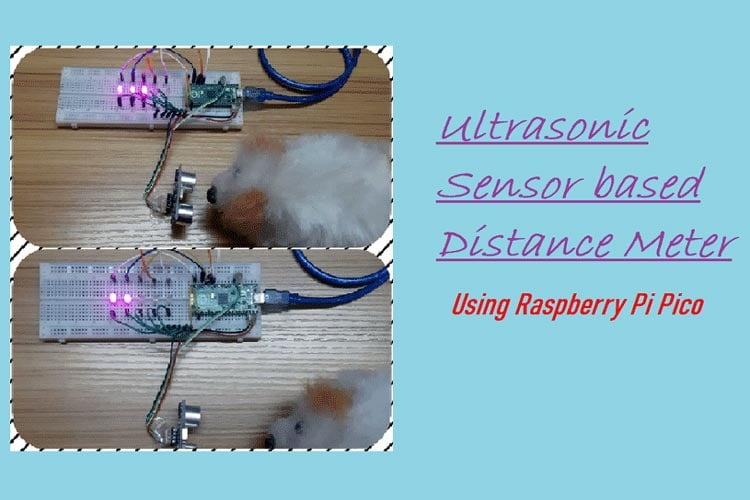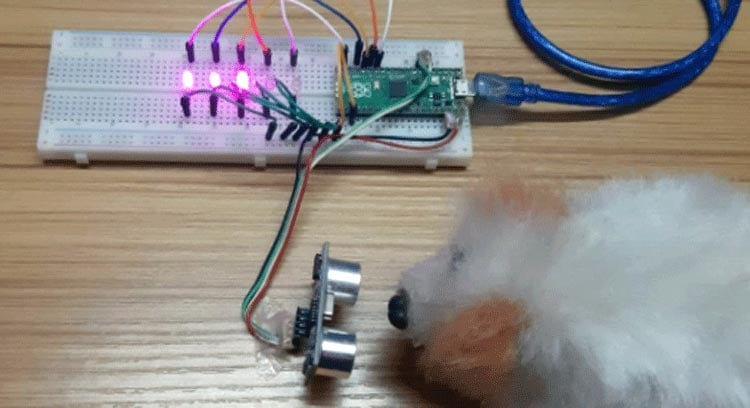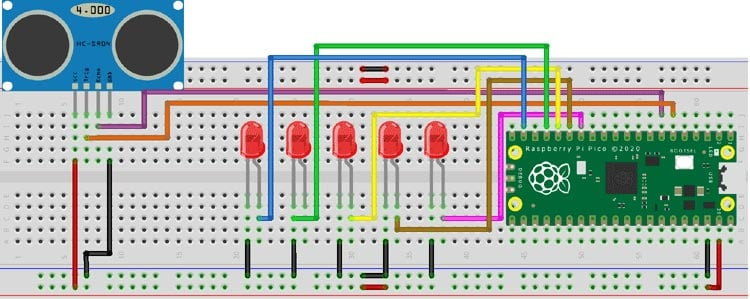# Build Raspberry Pi Pico based Ultrasonic Distance Meter

Published  February 28, 2022   0
I
AuthorThe distance meter is used for accurately determining the distance of an object without contact by the medium of ultrasonic sound waves(20,000 Hz above sound frequency). This raspberry pi pico based project will become a very important instrument in practical if it is used perfectly. In case we use a laser range finder it will not be efficient enough since laser beams will pass through a transparent medium(object) that will not be able to detect it. So this is way more efficient. Also, this project can be used in cars so as to detect any obstacles while parking. Or check the distance left to park safe fully.

Previously we built some distance measuring projects, here are some links:### Component Requirement for Distance Meter

Project Used Hardware

• Raspberry Pi Pico,
• Ultrasonic sensor,
• LEDs,
• Jumper wires

Project Used Software

• Thonny IDE

### Project Hardware Software Selection

I have used the Raspberry Pi Pico because it is a very small, low cost microcontroller board. Also, this board is much faster than any other small microcontroller board like Arduino Nano, or Arduino Pro Mini since it has a clock frequency of 133 MHz I have also chosen the ultrasonic sensor because it is more efficient in comparison to Laser Finder. LED to detect the approximate distance. I have used Thonny IDE to program the Raspberry Pi Pico using (Micro Python). Micro Python requires very small codes in comparison to Arduino IDE.

### Circuit DiagramHere in the Pico board, I have reserved 5 GPIO pins for 5 LEDs, 2 GPIO pins for the Trigger and Echo pin of the ultrasonic sensor. GPIO pin 6 and 7 are for the Trigger and Echo pins, respectively. GPIO pin 10, 11, 12, 13, and 14 are for the LEDs. The negative terminal of all LEDs and the ultrasonic sensor are connected together with the Ground pin of the Pico and the VCC pin of the sensor is connected to 5v of the Pico. The LEDs from GPIO pin 10 to 14 will start to glow gradually as the distance between the object and the sensor decreases(i.e. when the object comes closer).

Code
```# Ultrasonic Distance Meter using Raspberry Pi Pico

#Micro Python

from machine import Pin,PWM #importing PIN and PWM

import time #importing time

import utime

#Defining LED pins

LED1=Pin(10,Pin.OUT)

LED2=Pin(11,Pin.OUT)

LED3=Pin(12,Pin.OUT)

LED4=Pin(13,Pin.OUT)

LED5=Pin(14,Pin.OUT)

#Defining  Trigger and Echo pins

trigger = Pin(4, Pin.OUT)

echo = Pin(5, Pin.IN)

# Defining function to get distance from ultrasonic sensor

def get_distance():

trigger.low()

utime.sleep_us(2)

trigger.high()

utime.sleep_us(5)

trigger.low()

while echo.value() == 0:

signaloff = utime.ticks_us()

while echo.value() == 1:

signalon = utime.ticks_us()

timepassed = signalon - signaloff

dist = (timepassed * 0.0343) / 2

return dist

while True:

distance=get_distance() #Getting distance in cm

print(+distance)

#Defining LED ON and OFF based on conditions

if distance <=3:  #If distance is less or equal to 3cm

LED1.high()

LED2.high()

LED3.high()

LED4.high()

LED5.high()

elif distance <=5:  #If distance is less or equal to 5cm but greater than 3cm

LED1.low()

LED2.high()

LED3.high()

LED4.high()

LED5.high()

elif distance <=7:  #If distance is less or equal to 7cm but greater than 5cm

LED1.low()

LED2.low()

LED3.high()

LED4.high()

LED5.high()

elif distance <=10:  #If distance is less or equal to 10cm but greater than 7cm

LED1.low()

LED2.low()

LED3.low()

LED4.high()

LED5.high()

elif distance ==15: #If distance is equal to 15cm

LED1.low()

LED2.low()

LED3.low()

LED4.low()

LED5.high()

elif distance >15: #If distance is greater than 15cm

LED1.low()

LED2.low()

LED3.low()

LED4.low()

LED5.low()

```
Video

Tags